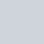# Statistical Inference Definiton, Types and Estimation Procedures in statistics

Inference comes from a word ‘infer’ which means that to draw a conclusion about a phenomenon on the basis of some information at hand. To make inference about a phenomenon through some statistical procedure is called statistical inference. According to wikipedia

“Inferences are steps in reasoning, moving from premises to logical consequences”.

## Types of statistical inference

Statistical inference may be divided into two categories. They are-

• Inductive Inference
• Deductive Inference

### Inductive statistical Inference

Inductive inference refers to draw a conclusion about a small part of the phenomenon and generalized it for the whole phenomenon. The research worker performs an experiment and obtain some data on the basis of the data. Certain conclusions are drawn and it is generalized for whole population. For example

### Deductive statistical Inference

By deductive inference we mean to draw a conclusion about a small part of phenomenon from the whole known phenomenon. For example, Let the distribution of population is known. We want to know the distribution of small part (sample) of the population (sampling distribution), then it is a problem of deductive inference. In this process we use the following terms-

• Estimator: Estimator is a function by which we find the value of estimate. Practically we can say that estimator is a material of finding an estimate.
• Estimate: Estimate is a value of those function which are used as estimator. Practically we can say that estimate is a value of the materials or estimator.
• Estimation: The process of finding an estimate of an estimator is called estimation. Practically we can say that estimation is a procedure of finding an estimate.

Basically, there are two parts exists in a statistical inference. They are-

• Estimation
• Test of hypothesis

Generally, the estimation is divided into two categories. They are-

• Point estimation
• Interval estimation

### Methods of point estimation

Some important methods of inferential point estimation are given below-

• Methods of moments
• Methods of maximum likelihood estimation
• Methods of minimum chi-square
• Methods of least square
• Bayesian Method
• Methods of minimum distance

### Properties of a good estimator

For a good estimator in the sense that the distribution of the estimator is concentrated near the true value of the parameter. The following properties have been developed-

• Unbiasedness
• Consistency
• Efficiency or minimum variance
• Minimum variance bound
• Sufficiency
• Mean square error etc

### Unbiasedness of an estimator

Let, ‘tn’ be any estimator or statistic calculated from a sample of size n drawn from any population of f(x; ϴ). If for every value of ϴ and x, E[tn]= ϴ; then tn is said to be unbiased estimator of ϴ. Otherwise tn is said to be biased estimator of ϴ. Biased of an estimator is measured by using the difference, that is B(t)= E[tn]- ϴ.

• If the amount of bias= positive=upward bias.
• If the amount of bias= negative= downward bias.
• If the amount of bias= 0 = unbiased.

### Consistency of an estimator

In statistics, an estimator tn is said to be a consistent estimator of the population parameter ϴ if tn converges statistically or in probability to ϴ as n→∞. Consistency is a large sample property which implies that a consistent estimator approaches to corresponding parameter in large sample. For example- sample mean, sample variance, sample moments are the consistent estimator of population mean, population variance and population moments respectively.

### Sufficiency of an estimator

An estimator is said to be sufficient estimator of population parameter, if it gives maximum information about the unknown parameter ϴ, ϴ ϵ H [H is parameter space].

Try some fresh content:

### One thought on “Statistical Inference Definiton, Types and Estimation Procedures in statistics”

1.Fashion Styles

Today, I went to the beachfront with my children. I found a sea shell and gave it to my 4 year old daughter and said “You can hear the ocean if you put this to your ear.” She placed the shell to her ear and screamed. There was a hermit crab inside and it pinched her ear. She never wants to go back! LoL I know this is completely off topic but I had to tell someone!